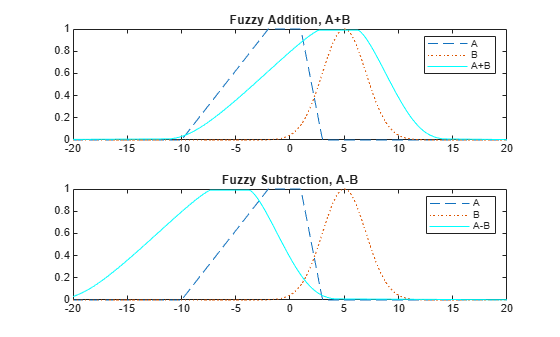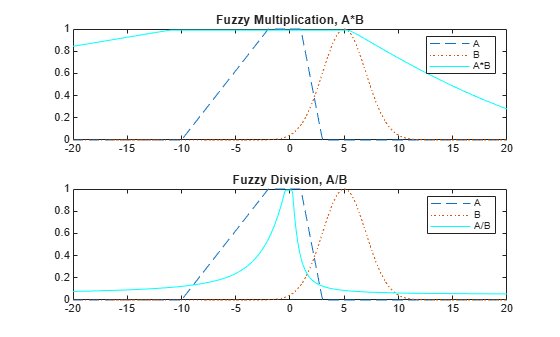# fuzarith

Perform fuzzy arithmetic

## Syntax

``C = fuzarith(X,A,B,operator)``

## Description

example

````C = fuzarith(X,A,B,operator)` returns the fuzzy set `C`, which is the result of applying the specified fuzzy operator to the fuzzy sets `A` and `B`. The operation is performed across the universe of discourse `X````

## Examples

collapse all

Specify Gaussian and trapezoidal membership functions.

```N = 501; minX = -20; maxX = 20; x = linspace(minX,maxX,N); A = trapmf(x,[-10 -2 1 3]); B = gaussmf(x,[2 5]);```

Evaluate the sum, difference, product, and quotient of `A` and `B`.

```Csum = fuzarith(x,A,B,'sum'); Csub = fuzarith(x,A,B,'sub'); Cprod = fuzarith(x,A,B,'prod'); Cdiv = fuzarith(x,A,B,'div');```

Plot the addition and subtraction results.

```figure subplot(2,1,1) plot(x,A,'--',x,B,':',x,Csum,'c') title('Fuzzy Addition, A+B') legend('A','B','A+B') subplot(2,1,2) plot(x,A,'--',x,B,':',x,Csub,'c') title('Fuzzy Subtraction, A-B') legend('A','B','A-B')```Plot the multiplication and division results.

```figure subplot(2,1,1) plot(x,A,'--',x,B,':',x,Cprod,'c') title('Fuzzy Multiplication, A*B') legend('A','B','A*B') subplot(2,1,2) plot(x,A,'--',x,B,':',x,Cdiv,'c') title('Fuzzy Division, A/B') legend('A','B','A/B')```## Input Arguments

collapse all

Universe of discourse, specified as a vector.

Input fuzzy set, specified as a vector with the same length as `X`. Each element of `A` is the value of the fuzzy set for the corresponding value of `X`.

`A` must be a convex fuzzy set. For more information, see Algorithms.

Input fuzzy set, specified as a vector with the same length as `X`. Each element of `B` is the value of the fuzzy set for the corresponding value of `X`.

`B` must be a convex fuzzy set. For more information, see Algorithms.

Arithmetic operator, specified as one of the following:

• `'sum'` — Fuzzy addition

• `'sub'` — Fuzzy subtraction

• `'prod'` — Fuzzy multiplication

• `'div'` — Fuzzy division

Note

Fuzzy addition can generate the message `"divide by zero"`. However, this warning does not affect the accuracy of `fuzarith`.

## Output Arguments

collapse all

Output fuzzy set, returned as a column vector with length equal to the length of `X`.

## Algorithms

To perform fuzzy arithmetic operations, the fuzzy operands (input fuzzy sets `A` and `B`) must be convex fuzzy sets. A fuzzy set is convex if, for each pair of points x1 and x2 in the universe of discourse `X` and λ∈[0,1].

`$\mu \left(\lambda {x}_{1}+\left(1-\lambda \right){x}_{2}\right)\ge \mathrm{min}\left(\mu \left({x}_{1}\right),\mu \left({x}_{2}\right)\right)$`

An α-cut of a fuzzy set is the region in the universe of discourse for which the fuzzy set has a specific membership value, α. For a convex fuzzy set, every α-cut defines a continuous region in the universe of discourse.

`fuzarith` uses the continuous regions defined by the α-cuts of fuzzy sets `A` and `B` to compute the corresponding α-cut of the output fuzzy set `C`. To do so, `fuzarith` uses interval arithmetic.

The following table shows how to compute the left and right boundaries of the output interval. Here:

• [AL AR] is the interval defined by the α-cut of fuzzy set A.

• [BL BR] is the interval defined by the α-cut of fuzzy set B.

• [CL CR] is the interval defined by the α-cut of fuzzy set C.

Interval Arithmetic OperatorDefinition
`$\begin{array}{l}{C}_{L}={A}_{L}+{B}_{L}\\ {C}_{R}={A}_{R}+{B}_{R}\end{array}$`
Subtraction: C = A-B
`$\begin{array}{l}{C}_{L}={A}_{L}-{B}_{R}\\ {C}_{R}={A}_{R}-{B}_{L}\end{array}$`
Multiplication: C = A*B
`$\begin{array}{l}{C}_{L}=\mathrm{min}\left({A}_{L}\cdot {B}_{L},{A}_{L}\cdot {B}_{R},{A}_{R}\cdot {B}_{L},{A}_{R}\cdot {B}_{R}\right)\\ {C}_{R}=\mathrm{max}\left({A}_{L}\cdot {B}_{L},{A}_{L}\cdot {B}_{R},{A}_{R}\cdot {B}_{L},{A}_{R}\cdot {B}_{R}\right)\end{array}$`
Division: C = A/B
`$\begin{array}{l}{C}_{L}=\mathrm{min}\left(\frac{{A}_{L}}{{B}_{L}},\frac{{A}_{L}}{{B}_{R}},\frac{{A}_{R}}{{B}_{L}},\frac{{A}_{R}}{{B}_{R}}\right)\\ {C}_{R}=\mathrm{max}\left(\frac{{A}_{L}}{{B}_{L}},\frac{{A}_{L}}{{B}_{R}},\frac{{A}_{R}}{{B}_{L}},\frac{{A}_{R}}{{B}_{R}}\right)\end{array}$`